Question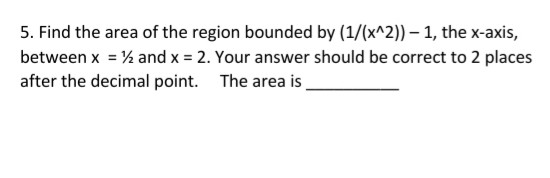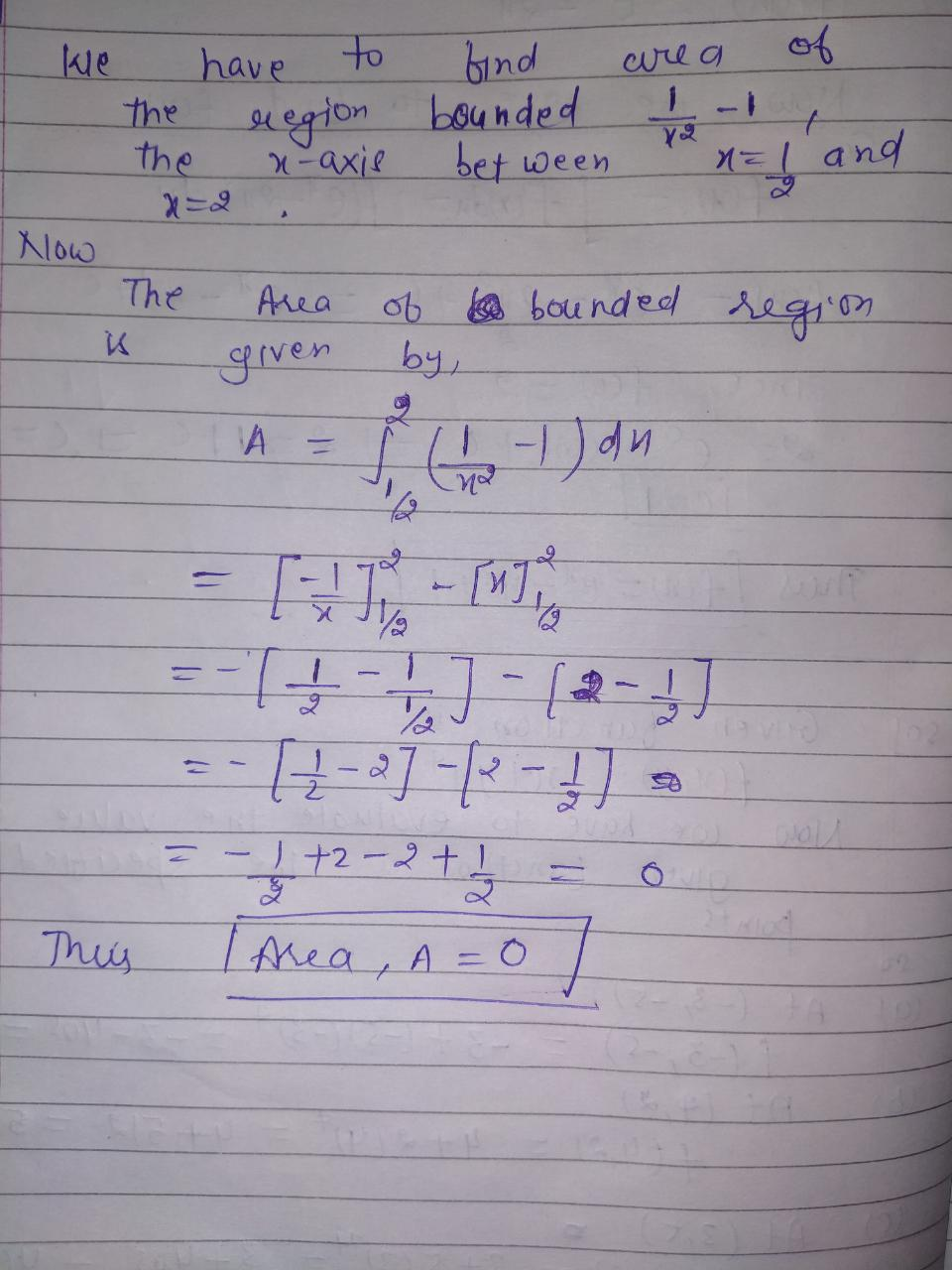#### Earn Coins

Coins can be redeemed for fabulous gifts.

Similar Homework Help Questions
• ### Find the net area and the area of the region bounded by y= 8 cos x and the x-axis between x--2 and x = π. Graph the function and find the region indicated in this question The net area is(Simplify yo...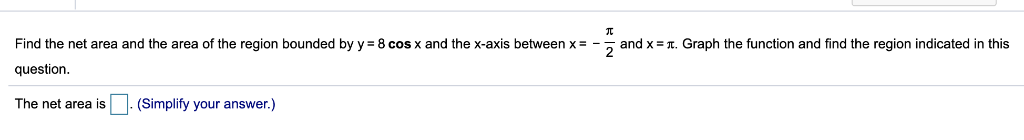Find the net area and the area of the region bounded by y= 8 cos x and the x-axis between x--2 and x = π. Graph the function and find the region indicated in this question The net area is(Simplify your answer.) Find the net area and the area of the region bounded by y= 8 cos x and the x-axis between x--2 and x = π. Graph the function and find the region indicated in this question The net...

• ### 4. Find the volume of the solid formed by rotating the region bounded by y =...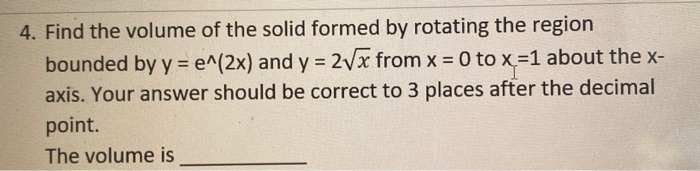4. Find the volume of the solid formed by rotating the region bounded by y = e^(2x) and y = 2Vx from x = 0 to x =1 about the x- axis. Your answer should be correct to 3 places after the decimal point. The volume is

• ### Find the area of the region bounded by the graph of f(x) = x(x + 1)(x...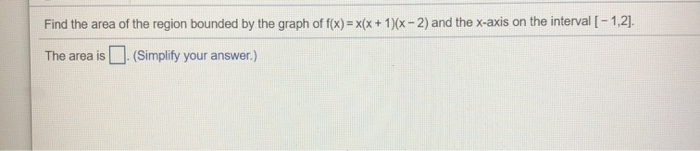Find the area of the region bounded by the graph of f(x) = x(x + 1)(x - 2) and the x-axis on the interval [ -1,2). The area is (Simplify your answer.)

• ### Math23 2 Consider the region in first quadrant area bounded by y x, x 6, and...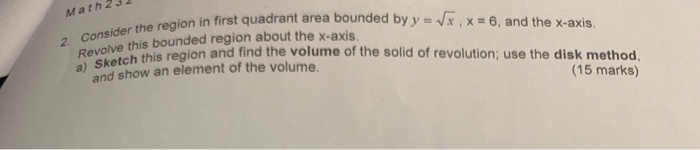Math23 2 Consider the region in first quadrant area bounded by y x, x 6, and the x-axis. Revolve this bounded region about the x-axis a) Sketch this region and find the volume of the solid of revolution; use the disk method and show an element of the volume. (15 marks) b) Find the coordinates of the centroid of the solid of revolution. c) Find the coordinates of the centroid of the plate; on the sketch above, show the vertical...

• ### Use the Midpoint Rule with n = 5 to approximate the area of the region bounded...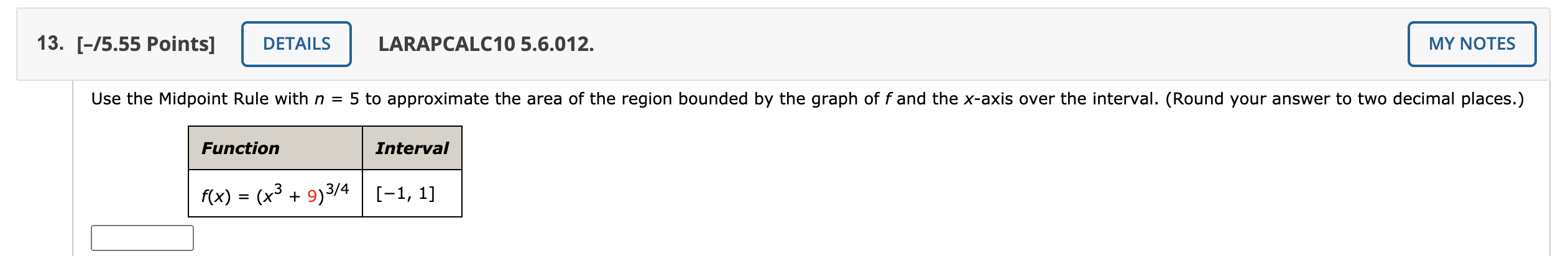Use the Midpoint Rule with n = 5 to approximate the area of the region bounded by the graph of f and the x-axis over the interval. (Round your answer to two decimal places.) 13. [-75.55 Points] DETAILS LARAPCALC10 5.6.012. MY NOTES Use the Midpoint Rule with n = 5 to approximate the area of the region bounded by the graph of f and the x-axis over the interval. (Round your answer to two decimal places.) Function Interval f(x) =...

• ### 3.Find the area of the region bounded by the parametric curve and the x-axis. (10 pts)...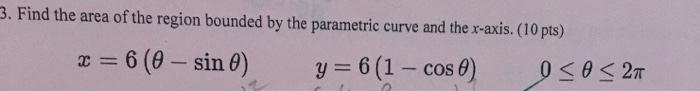3.Find the area of the region bounded by the parametric curve and the x-axis. (10 pts) = 6 (0- sin 0) y=6(1 - cos 0) 0<02T Find the slope of the tangent line at the given point. (10 pts) 4. r 2+sin 30, 0=T/4

• ### Find the area of the region bounded by the graph off and the x-axis on the...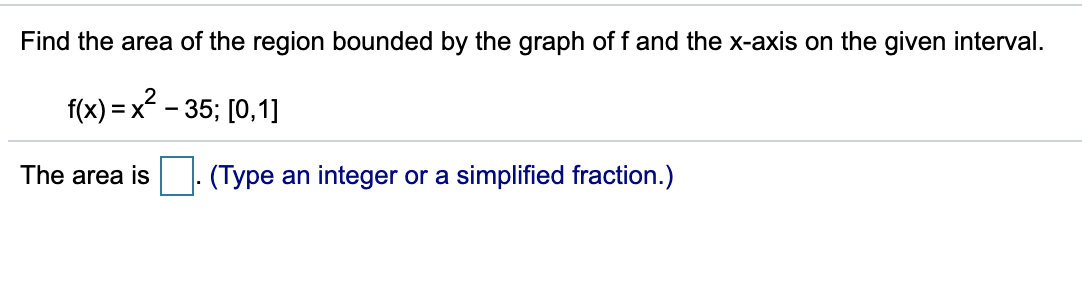Find the area of the region bounded by the graph off and the x-axis on the given interval. f(x) = x² – 35; [0,1] The area is (Type an integer or a simplified fraction.)

• ### Math232 2 Consider the region in first quadrant area bounded by y x,x=6, and the x-axis....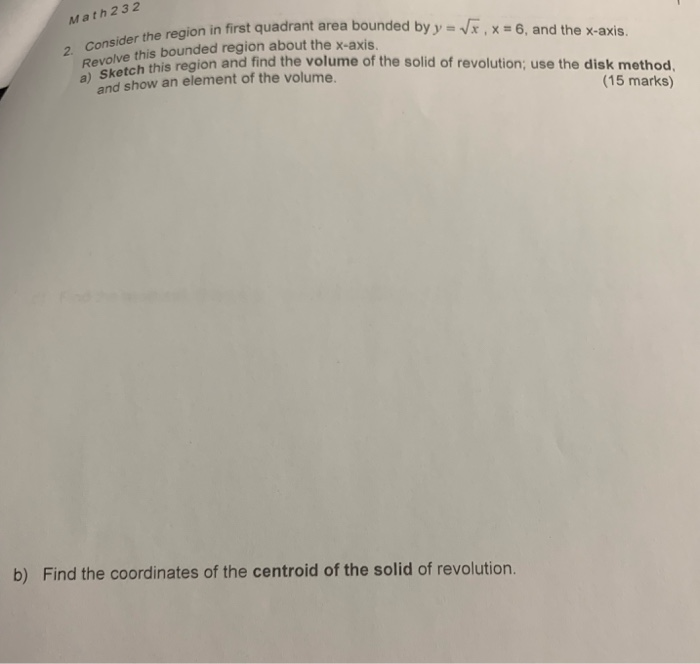Math232 2 Consider the region in first quadrant area bounded by y x,x=6, and the x-axis. Revolve this bounded region about the x-axis a) Sketch this region and find the volume of the solid of revolution; use the disk method, and show an element of the volume. (15 marks) b) Find the coordinates of the centroid of the solid of revolution Find the moment of inertia of the solid of revolution with respect to the x-axis. d) Math232 2 Consider...

• ### 5. (12 pts.) Consider the region bounded by f(x) 4-2x and the x-axis on interval [-1,...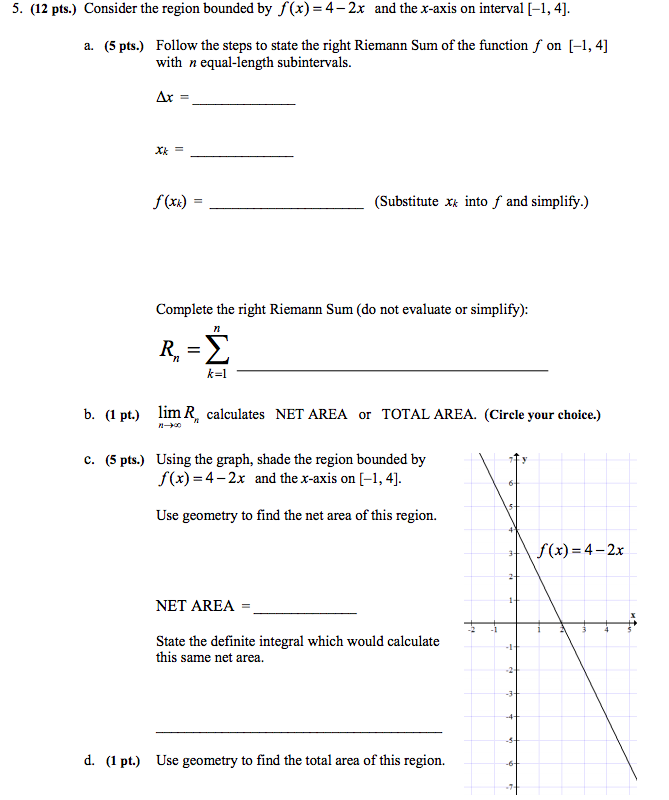5. (12 pts.) Consider the region bounded by f(x) 4-2x and the x-axis on interval [-1, 4] Follow the steps to state the right Riemann Sum of the function f with n equal-length subintervals on [-, 4] (5 pts.) a. Xk= f(xa) (Substitute x into f and simplify.) Complete the right Riemann Sum (do not evaluate or simplify): -2 b. (1 pt.) lim R calculates NET AREA or TOTAL AREA. (Circle your choice.) Using the graph, shade the region bounded...

• ### Find the area of the region between curves       1. Find Find the area of...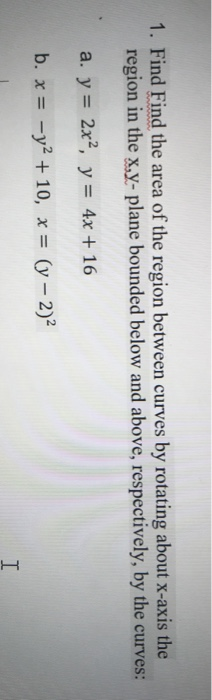Find the area of the region between curves       1. Find Find the area of the region between curves by rotating about x-axis the region in the x,y- plane bounded below and above, respectively, by the curves: a. y = 2x2, y = 4x + 16 b. x = -y2 + 10, x = (y – 2) I